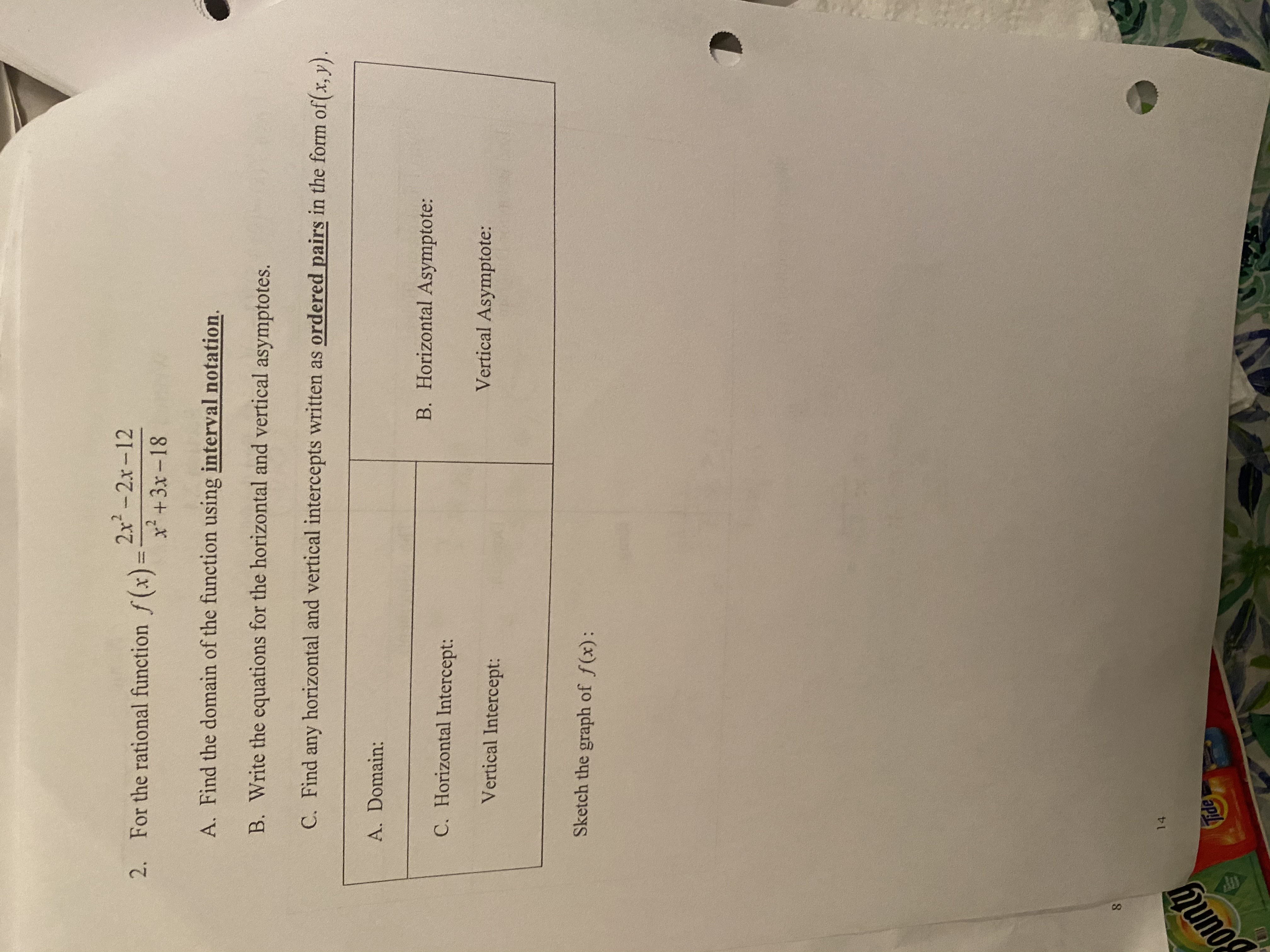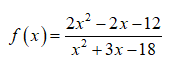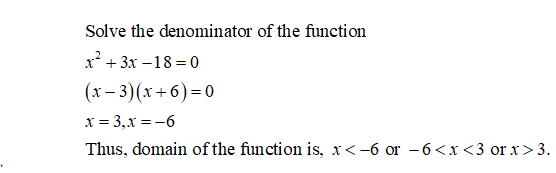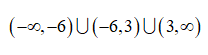# 2. For the rational function f(x)=2x2-2x-12%3Dx+3x-18A. Find the domain of the function using interval notation.B. Write the equations for the horizontal and vertical asymptotes.C. Find any horizontal and vertical intercepts written as ordered pairs in the form of(x, y).A. Domain:C. Horizontal Intercept:B. Horizontal Asymptote:Vertical Intercept:Vertical Asymptote:Sketch the graph of f(x):8.14ountyTide

Question
2 viewshelp_outlineImage Transcriptionclose2. For the rational function f(x)= 2x2-2x-12 %3D x+3x-18 A. Find the domain of the function using interval notation. B. Write the equations for the horizontal and vertical asymptotes. C. Find any horizontal and vertical intercepts written as ordered pairs in the form of(x, y). A. Domain: C. Horizontal Intercept: B. Horizontal Asymptote: Vertical Intercept: Vertical Asymptote: Sketch the graph of f(x): 8. 14 ounty Tide fullscreen
check_circle

Step 1
1. The given rational function is,Step 2

A. The domain of the given function is obtained as follows.Step 3

The domain of the function in the interval notation is,...

### Want to see the full answer?

See Solution

#### Want to see this answer and more?

Solutions are written by subject experts who are available 24/7. Questions are typically answered within 1 hour.*

See Solution
*Response times may vary by subject and question.
Tagged in